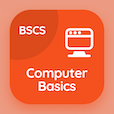Online CS Degree Courses

Database Management System MCQs

Database Management System MCQ - Topics

Practice UML Diagrams Multiple Choice Questions (MCQ Quiz), UML Diagrams quiz answers PDF to study database management system course for database management system online classes. Database Design Methodology and UML Diagrams Multiple Choice Questions and Answers (MCQs), UML Diagrams quiz questions for online software development courses. UML Diagrams Book PDF: unified modeling language diagrams, uml diagrams, information system life cycle, uml class diagrams test prep for online computer science schools.

"The activity diagram, use case diagram, collaboration diagram and sequence diagram are considered as types of" MCQ PDF: uml diagrams App APK with non-behavioral diagrams, non structural diagrams, structural diagrams, and behavioral diagrams choices for online software development courses. Learn uml diagrams quiz questions for merit scholarship test and certificate programs for computer software engineer.

## MCQ on UML Diagrams Quiz

MCQ: The activity diagram, use case diagram, collaboration diagram and sequence diagram are considered as types of

non-behavioral diagrams
non structural diagrams
structural diagrams
behavioral diagrams

MCQ: The kind of diagrams which are used to show interactions between series of messages are classified as

activity diagrams
state chart diagrams
collaboration diagrams
object lifeline diagrams

MCQ: The diagrams which are used to distribute files, libraries and tables across topology of hardware are called

deployment diagrams
use case diagrams
sequence diagrams
collaboration diagrams

MCQ: The dynamic aspects related to a system are shown with the help of

sequence diagrams
interaction diagrams
deployment diagrams
use case diagrams

### More Quizzes on Database Management System Book